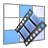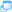# rubric gallery

## rubrics by sobakrishnanpublic gallery
 find rubricrubrics by sobakrishnan
See a list of rubrics by sobakrishnan. Copy rubrics to your zone. Bookmark rubrics for future use Build, share, exchange, and reuse rubrics. Find rubrics by category and type.

Click on rubric title to preview, edit, print, copy, or do more with it.Quickly preview a rubric in a popup window.
Found 21 Rubrics   (showing Rubrics 1 thru 20 )
21
Rubrics
Title      Built By
1Circular MeasureLearning Outcome: Students will be able to solve problems involving circular measure.

sobakrishnan
2Add Math Problem Solving Rubricto assessment student's performance for a given task

sobakrishnan
3Add Math Problem Solving Rubricto assessment student's performance for a given task

sobakrishnan
4Vectors and Relative VectorsLearning Outcome: Students will be able to solve problems involving vectors and relative vectors.

sobakrishnan
5Remainder and Factor TheoremLearning Outcome: Students will be able to apply factor and remainder theorem to solve equations.

sobakrishnan
6KinematicsLearning Outcome: Students will be able to solve problems involving displacement, velocity and acceleration.

sobakrishnan
7Integration and application of IntegrationLearning Outcome: Students will be able to integrate functions and apply the knowledge to find the areas of the regions.

sobakrishnan
8Derivatives of Trigonometric, Exponential and Log FunctionsLearning Outcome: Students will be able to differentiate trigonometric, exponential and logarithmic function.

sobakrishnan
9Differentiation and its applicationsLearning Outcome: Students will be able to differentiate the function and apply in problem solving

sobakrishnan
10Trigonometric Functions and IdentitiesLearning Outcome: Students will be able to sketch graphs of trigonometric functions and also apply the identitie

sobakrishnan
11Quadratic Expressions and EquationsLearning Outcome: Students will be able to solve problems involving quadratic functions or equations.

sobakrishnan
12Simultaneous EquationsLearning Outcome: Students will be able to solve simultaneous equations.

sobakrishnan
13MatricesLearning Outcome: Students will be able to do basic operations with matrices and also solve simultaneous equation using matrix method.

sobakrishnan
14Permutations and CombinationsLearning Outcome: Students will be able to recognise and distinguish between a permutation case and a combination case. answer simple problems on arrangement and selection (cases with repetition of objects, or with objects arranged in a circle or involving both permutations and combinations, are excluded)

sobakrishnan
15Binomial TheoremLearning Outcome: Students will be able to use binomial theorem to expand and also find the coefficient for a particular term.

sobakrishnan
16Circular MeasureLearning Outcome: Students will be able to solve problems involving circular measure.

sobakrishnan
17Coordinate GeometryLearning Outcome: Students will be able to solve problems involving coordinate geometry

sobakrishnan
18Indices, Surds and LogarithmsLearning Outcome: Students will be able to solve problems involving indices, surds and logarithms

sobakrishnan
19Set Language and NotationLearning Outcome: Students will be able to use set language and notation, and Venn diagrams to describe sets and represent relationships between sets

20Functions